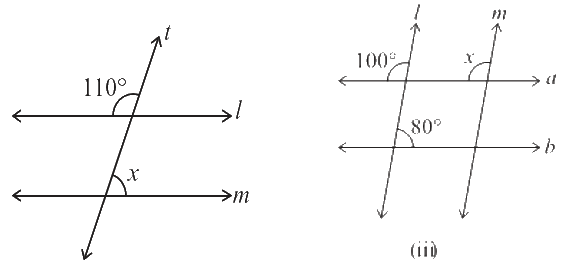# Ex.5.2 Q4 Lines and Angles - NCERT Maths Class 7

Go back to  'Ex.5.2'

## Question

Find the value of $$x$$ in each of the following figures if  $$l || m.$$Video Solution
Lines & Angles
Ex 5.2 | Question 4

## Text Solution

(i) Reasoning:

There are two operations done in sequence. First, find the corresponding angle to $$110^\circ$$ i. e $$\angle y,$$ then by using Linear pair find the value of $$\angle x.$$

According to this model, the result $$\angle x + \angle y = 180^\circ.$$ Now, it’s a matter of finding value of $$\angle x.$$

(i) Steps:

Solve for $$x$$

Given $$l || m$$ and $$t$$ is transversal,

\begin{align}\angle y &= 110^\circ\text{(Corresponding angle)}\\ \angle x+\angle y &= 180^\circ\text{(Linear pair)}\\\angle x &= 180^\circ - 110^\circ\\\angle x &= 70^\circ\end{align}

(ii) Reasoning:

Let’s visually model this problem. There is one operation that can be done. Find the corresponding angle to $$x.$$ According to this model, the resultant value of corresponding angle will be equal to $$x.$$ Now, it’s a matter of finding measure of $$x.$$

(ii) Steps:

Solve for $$x$$

Given $$l || m$$ and $$a || b,$$

$\angle x = 100^\circ \text{(corresponding angle)}$

Learn from the best math teachers and top your exams

• Live one on one classroom and doubt clearing
• Practice worksheets in and after class for conceptual clarity
• Personalized curriculum to keep up with school